RoboTHOR comes packaged with AI2-THOR, which can be installed in Python using pip:pip install ai2thor

After installing AI2-THOR, we can initialize a controller, which will allow us to execute actions in the environment.from ai2thor.controller import Controller

controller = Controller(
agentMode="locobot",
visibilityDistance=1.5,
scene="FloorPlan_Train1_3",
gridSize=0.25,
movementGaussianSigma=0.005,
rotateStepDegrees=90,
rotateGaussianSigma=0.5,
renderDepthImage=False,
renderInstanceSegmentation=False,
width=300,
height=300,
fieldOfView=60
)

### Controller Parameters

Always set this to

locobot
in order to use the LoCoBot agent, which was designed for RoboTHOR.

Warning
If unset, the agent will be the default iTHOR agent, which is not the LoCoBot, and thus has different properties than these docs.

A RuntimeError is raised if

agentMode
is not set to "default", "locobot", "drone", or "arm".

Used to set the maximum goal distance in meters between the agent and an object. This affects each object's visible property, where when

True
, the agent is within
visibilityDistance
of that object and the object appears in the agent's current egocentric frame.

Remark
Visibility is defined on iTHOR's concepts page.

The name of which scene to initialize. Valid RoboTHOR scenes are in the form

FloorPlan_Train{1:12}_{1:5}
or
FloorPlan_Val{1:3}_{1:5}
, such as
FloorPlan_Train3_5
or
FloorPlan_Val1_2
.

The mean amount, in meters, that the agent moves after calling a move action (i.e.,

and
MoveBack
). The full amount that the agent moves is sampled from $\mathcal N(\mu=\texttt{gridSize}, \sigma=\texttt{movementGaussianSigma})$.

The standard deviation, in meters, controlling the amount of noise in a move action (i.e.,

and
MoveBack
). The full amount that the agent moves is sampled from $\mathcal N(\mu=\texttt{gridSize}, \sigma=\texttt{movementGaussianSigma})$.

The mean amount, in degrees, that the agent rotates after calling a rotate action (i.e.,

RotateLeft
or
RotateRight
). The full amount that the agent rotates is then sampled from $\mathcal N(\mu=\texttt{rotateStepDegrees}, \sigma=\texttt{rotateGaussianSigma})$.

The standard deviation, in degrees, controlling the amount of noise in a rotate action (i.e.,

RotateLeft
or
RotateRight
). The full amount that the agent rotates is then sampled from $\mathcal N(\mu=\texttt{rotateStepDegrees}, \sigma=\texttt{rotateGaussianSigma})$.

When

True
, a depth frame is rendered and made available as an event.depth_frame attribute.

We require this to be explicitly passed in because rendering depth takes longer than rendering RGB alone.

When

True
, a instance segmentation frame is rendered and made available as an
Event.instance_segmentation_frame
attribute. Instance segmentation provides segmentation by object instance, where all objects in view are distinguishable.

We require this to be explicitly passed in because rendering instance segmentation takes longer than rendering RGB alone.

The number of horizontal sampled pixels for each frame. This affects every rendered image frame (e.g., RGB, depth, BGR).

The number of vertical sampled pixels for each frame. This affects every rendered image frame (e.g., RGB, depth, BGR).

Changes the camera's optical field of view, in degrees. Valid values are in the domain $(0:180)$. The default field of view for the LoCoBot is 60 degrees.

Remark

Not all agent modes have the same default field of view. For instance, the drone's field of view is 150 degrees, by default.

Any

Initialization parameter can later be changed by calling the reset method. For instance, we can call:controller.reset(scene="FloorPlan_Train7_5", rotateStepDegrees=30)

The values will default to what they were upon the most recent reset or initialization. For instance, if you initialized with

fieldOfView=45
, then called
reset
with
fieldOfView=60
, calling
reset
again, without passing in
fieldOfView
will keep
fieldOfView=60
.

The movement and rotation action mimic what is possible from a physical LoCoBot. We also provide the ability to

Teleport
and query the environment with
GetReachablePositions
.

Since the LoCoBot can only

and
MoveBack
in real life, its simulated counterpart does not support actions like
MoveLeft
and
MoveRight
.controller.step(
moveMagnitude=0.25
)

# Other supported direction
controller.step("MoveBack")

### Movement Parameters

Deterministically overrides the initialized step size that is defaulted to sample from $\mathcal N(\mu=\texttt{gridSize}, \sigma=\texttt{movementGaussianSigma})$. The units are specified in meters.

Changes the yaw rotation of the agent.controller.step(
action="RotateRight",
degrees=90
)

# Other supported direction
controller.step("RotateLeft")

### Agent Rotation Parameters

Deterministically overrides the initialized rotation increment without noise. Defaults to sampling from $\mathcal N(\mu=\texttt{rotateStepDegrees}, \sigma=\texttt{rotateGaussianSigma})$

We can adjust the camera's pitch by utilizing the

LookUp
and
LookDown
commands. Both increment in $30^\circ$ intervals, with angles being clamped between $30^\circ$ in the downward direction and $30^\circ$ in the upward direction.controller.step("LookUp")
controller.step("LookDown")

Teleport
allows the agent to set its pose to anywhere in the scene in a single step. Valid poses do not place the agent outside the outer boundaries of the scene or in an area that it collides with an object.controller.step(
action="Teleport",
position=dict(x=0.999, y=1.01, z=-0.3541),
rotation=dict(x=0, y=90, z=0),
horizon=30
)

### Teleport Parameters

The global position of the agent, with keys for $x$, $y$, and $z$.
If
position
is unspecified, the agent's position will remain unchanged.
The coordinate space for each RoboTHOR scene is shown below. Notice that $y$ corresponds to the upward coordinate in 3D space.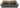The rotation of the agent's body in global 3D space. Here, the rotation changes the agent's yaw rotation.

If

rotation
is unspecified, the agent's rotation will remain unchanged.

Remark

The LoCoBot's body cannot changes its in pitch or roll rotations; hence, why the $x, z=0$.

The

horizon
change's the camera's rotation. Values are clamped between $[-30:30]$.

If

horizon
is not specified, the horizon will remain unchanged.

Since the agent looks up and down in $30^\circ$ increments, it most common for the horizon to be in $\lbrace -30, 0, 30\rbrace$.

Warning

As illustrated in the figure below, negative camera

horizon
values correspond to agent looking up, whereas positive
horizon
values correspond to the agent looking down.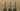In addition to being able to

Teleport
, we also have an equivalent action
TeleportFull
that strictly requires every degree of freedom to be specified. The strictness of this action is often useful in large projects, where we don't want to any part of the agent implied implicitly.

Warning

TeleportFull
does not guarantee backwards compatibility in future releases. If a new degree of freedom is added to the agent, it will be added as a required parameter. If this is an issue, we recommend using
Teleport
.controller.step(
action="TeleportFull",
position=dict(x=0.999, y=1.01, z=-0.3541),
rotation=dict(x=0, y=90, z=0),
horizon=30
)

### TeleportFull Parameters

The global position of the agent, with keys for $x$, $y$, and $z$.
If
position
is unspecified, the agent's position will remain unchanged.
The coordinate space for each RoboTHOR scene is shown below. Notice that $y$ corresponds to the upward coordinate in 3D space.The rotation of the agent's body in global 3D space. Here, the rotation changes the agent's yaw rotation.

The LoCoBot's body cannot change in pitch or roll; hence, why the $x, z=0$.

The

horizon
change's the camera's rotation. Values are clamped between [-30:30].

Since the agent looks up and down in $30^\circ$ increments, it most common for the horizon to be in $\lbrace -30, 0, 30 \rbrace$.

Warning

As illustrated in the figure below, negative camera

horizon
values correspond to agent looking up, whereas positive
horizon
values correspond to the agent looking down.It is often useful to randomize the position of the agent in the agent in the scene, before starting an episode. Here, we can use:

1. GetReachablePositions
, which does an optimized BFS over the in a deterministic grid spaced out by the initialized
gridSize
. The valid positions are then added and returned in a list.
2. Teleport
, which can take a given position, and transform our agent to that position.

The process is illustrated below:

Step 1: Get the Positionspositions = controller.step(
action="GetReachablePositions"
).metadata["actionReturn"]
Response
[
dict(x=(...), y=(...), z=(...)),
dict(x=(...), y=(...), z=(...)),
dict(x=(...), y=(...), z=(...)),
{...}
dict(x=(...), y=(...), z=(...)),
]

Step 2: Teleport to a Positionimport random
position = random.choice(positions)
controller.step(
action="Teleport",
position=position
)

### GetReachablePositions Response

A list of each position that the agent can be at, without colliding into any other objects or going beyond the walls.

The coordinate space for each RoboTHOR scene is shown below. Notice that $y$ corresponds to the upward coordinate in 3D space.The

Done
action nothing to the state of the environment. But, it returns a cleaned up event with respect to the metadata.controller.step(action="Done")

It is often used in the definition of a successful navigation task (see Anderson et al.), where the agent must call the done action to signal that it knows that it's done, rather than arbitrarily or biasedly guessing.

Warning

The

Done
action does literally nothing to the state of the environment. For instance, if the agent calls
Done
and then
, the
Done
action will have no affect on preventing a
action from executing.

It is often used to return a cleaned up version of the metadata.The figure above shows AI2-THOR's agent-simulator interaction loop. Our backend scenes, actions, and observations are stored within Unity, a powerful real-time game engine.

From the Python side, we sequentially interact with Unity by taking actions using AI2-THOR's Python

Controller
. We have already seen several actions, such as
,
RotateRight
, and
LookUp
.

The returned data after executing any action is an

Event
. Each
Event
contains a ton of information to encode the state of the environment after the action has been executed:event = controller.step(...)
References the last executed Eventcontroller.last_event
Response
<ai2thor.server.Event

.frame: {...}
.cv2img: {...}

.depth_frame: {...}

.instance_segmentation_frame: {...}
.instance_detections2D: {...}

.color_to_object_id: {...}
.object_id_to_color: {...}
/>


### Event Response

Contains information about the action and how it affected the environment. See the Metadata section for more.

RGB frame of the scene from the agent egocentric perspective. The size of the frame is (height, width, 3) stored in the numpy.uint8 format.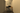Same as the frame, except image channels are in BGR ordering. This is often useful with Python's OpenCV module (i.e., cv2), which expects images with BGR orderings.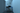Measures the distance, in meters, from the agent to each pixel in the frame. The size of the frame is (height, width) stored in the numpy.float32 format.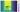Remark

Upon initialization or reset, renderDepthImage must be set to

True
in order for the depth frame to appear.

Segments every object instance in the scene. The size of the frame is (height, width, 3) stored in the numpy.uint8 format. Each unique pixel color corresponds to a different object, which are indexable with color_to_object_id or object_id_to_color.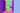Remark

Upon initialization or reset, renderInstanceSegmentation must be set to

True
in order for the instance segmentation frame to appear.

Segments every object instance in the scene, storing each segmentation as a boolean image mask.

The keys are object IDs visible in the frame and each value is the boolean numpy.ndarray of sizeheight, width) that segments the image.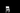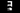Remark

Upon initialization or reset, renderInstanceSegmentation must be set to

True
in order for the instance masks to appear.

Provides the 2D bounding box around every object instance in the scene.

The keys are object IDs and the values are [Upper Left $x$, Upper Left $y$, Lower Right $x$, Lower Right $y$], where each element is the number of pixels it is from the top left corner of the image.

Remark

Upon initialization or reset, renderInstanceSegmentation must be set to

True
in order for the 2D instance detections to appear.

Provides a mapping from each pixel's color, represented as a hashable tuple, in the instance_segmentation_frame to what object it represents.

The metadata dictionary contains summary information about the state of the environment.event.metadata
Response
{
"errorMessage": {...}
"lastActionSuccess": {...}
"actionReturn": {...}

"agent": {...}
"objects": {...}

{...}
}


If an action fails, feedback is provided as to what caused the failure.

States whether the last action was able to successfully execute.

An exception will not be raised upon invalid states. For instance, if an agent is standing right in-front of a wall and tries to

, it will fail, but an exception will not be raised.

If an action is unsuccessful, the state of the environment will not have changed.

Holds the resulting data for actions that query the environment, such as Get Reachable Positions.

Within the metadata dictionary, the agent's key contains the pose of the agent after the action has executed.event.metadata["agent"]
Response
{
cameraHorizon: {...},
position: {...},
rotation: {...},
{...}
}


The angle in degrees that the camera's pitch is rotated.

Warning

As illustrated in the figure below, negative camera

horizon
values correspond to agent looking up, whereas positive
horizon
values correspond to the agent looking down.The global position of the agent, with keys for $x$, $y$, and $z$.

The coordinate space for each RoboTHOR scene is shown below. Notice that $y$ corresponds to the upward coordinate in 3D space.The local rotation of the agent's body, with keys for $x$ (pitch), $y$ (yaw), and $z$ (roll). Since the LoCoBot's body can only change its yaw rotation, both $x$ and $z$ will always be approximately 0.event.metadata["objects"]
Response
{
"objectId": {...},
"objectType": {...}

"distance": {...},
"visible": {...},

"position": {...},
"rotation": {...},

{...}
}


The unique ID of each object in the scene. It is generated at runtime and composed of an object's

objectType
and
position
.

Example:

AlarmClock|-02.08|+00.94|-03.62
.

The annotated type of the object. Each time is specified in the Object Types section.

The Euclidean distance from near the center-point of the object to the agent's camera.

Indicates whether the object is visible, and within the initialized visibility distance of the agent.

Warning

The visible property does not mean the object is literally visible in the frame. Rather, the object both has to be visible and at a distance of less than visibilityDistance away. For more information, refer to iTHOR's concepts page.

The global position of the object, with keys for $x$, $y$, and $z$.

The coordinate space for each RoboTHOR scene is shown below. Notice that $y$ corresponds to the upward coordinate in 3D space.The local rotation of the object, with keys for $x$ (pitch), $y$ (yaw), and $z$ (roll).

This section details several utility functions that may help when evaluating navigation agents in RoboTHOR. We also provide all object types that appear in RoboTHOR. For ObjectNav, object types often appear as navigation target.TARGET_OBJECT_TYPES = {
"AlarmClock,"
"Apple,"
"BaseballBat,"
"Bowl,"
"GarbageCan,"
"HousePlant,"
"Laptop,"
"Mug,"
"RemoteControl,"
"SprayBottle,"
"Television,"
"Vase"
}

### Target Object Types

Target object types are guaranteed to have exactly one instance of that type in each scene. Thus, they are well suited to use as target objects in an ObjectNav task.BACKGROUND_OBJECT_TYPES = {
"ArmChair",
"Bed",
"Book",
"Bottle",
"Box",
"ButterKnife",
"Candle",
"CD",
"CellPhone",
"Chair",
"CoffeeTable",
"Cup",
"DeskLamp",
"Desk",
"DiningTable",
"Drawer",
"Dresser",
"FloorLamp",
"Fork",
"Newspaper",
"Painting",
"Pencil",
"Pen",
"PepperShaker",
"Pillow",
"Plate",
"Pot",
"SaltShaker",
"Shelf",
"SideTable",
"Sofa",
"Statue",
"TeddyBear",
"TennisRacket",
"TVStand",
"Watch"
}

### Background Object Types

Background object types are then defined as the set of object types that are not target objects. Put differently, these objects appear in at least 1 scene, but do not appear in all of them.

We can use

get_shortest_path_to_object_type
to get the shortest path to a target object type. It returns a list of positions, representing the corner points on the path.from ai2thor.util.metrics import (
get_shortest_path_to_object_type
)

path = get_shortest_path_to_object_type(
controller=controller,
object_type="Apple",
initial_position=dict(
x=0,
y=0.9,
z=0.25
)
)
Response
[
dict(x={...}, y={...}, z={...}),
dict(x={...}, y={...}, z={...}),
dict(x={...}, y={...}, z={...}),
{...}
dict(x={...}, y={...}, z={...}),
]


### get_shortest_path_to_object_type Parameters

An initialized AI2-THOR

Controller
object.

The name of the target object type. A

ValueError
is thrown if
object_type
is not a target object type.

The starting position of the agent. Positions can be accessed from

.

We can use

path_distance
to compute the summation over all the edges in our
path
. An edge's value is defined as the L2 norm between 2 consecutive corner points.from ai2thor.util.metrics import (
path_distance
)

path_distance(path)

### path_distance Parameters

A list of dictionaries with keys for $x$, $y$, and $z$ agent positions along the path. This may also be the response from Shortest Path, for instance.

SPL, Success weighted by (normalized inverse) Path Length, is a quick and common navigation metric (see Anderson et al. and Batra et al.) as

$\text{SPL}_i = S_i \cdot \frac{\ell_i}{\max(p_i, \ell_i)},$

where, for a single episode $i$, $S_i$ is the binary indicator variable denoting if the episode was successful, $\ell_i$ is the shortest path length (in meters) from the agent's starting position to the target, and $p_i$ is the path length (in meters) that the agent took. The metric ranges inclusively from $[0:1]$.

Remark

It may look weird that we compute $\max(p_i, \ell_i)$. Since $p_i$ is defined as the shortest path length, one may think $\max(p_i, \ell_i) = \ell_i$ for all paths $\ell_i$. But, $p_i$ is defined as the shortest path length directly to the object, without the minimum cushion of distance, whereas for the agent's path, $\ell_i$, it may act optimally and then stop, say, half a meter short of the target with the episode still be considered successful. So, here, we'd have $\ell_i \gt p_i$.from ai2thor.util.metrics import (
compute_single_spl
)

compute_single_spl(
path,
shortest_path,
successful_path
)

### compute_single_spl Parameters

The $\ell_i$ term in the SPL definition.

A list of dictionaries with keys for $x$, $y$, and $z$ agent positions along the path.

The $p_i$ term in the SPL definition.

For ObjectNav, it is most easily calculated using the Shortest Path utility.

The $S_i$ term in the SPL definition.

For instance, with ObjectNav, we often define an episode of navigation to be successful if all of the following criteria are met:

1. The agent terminates the episode by issuing the
DONE
action.
2. The specified object is within a distance of 1 meter from the agent's camera.
3. The object is within the viewport of the agent.
Remark

We can use the visible object metadata property along with initializing visibilityDistance to 1 meter, to ensure that (2) and (3) have been satisfied.